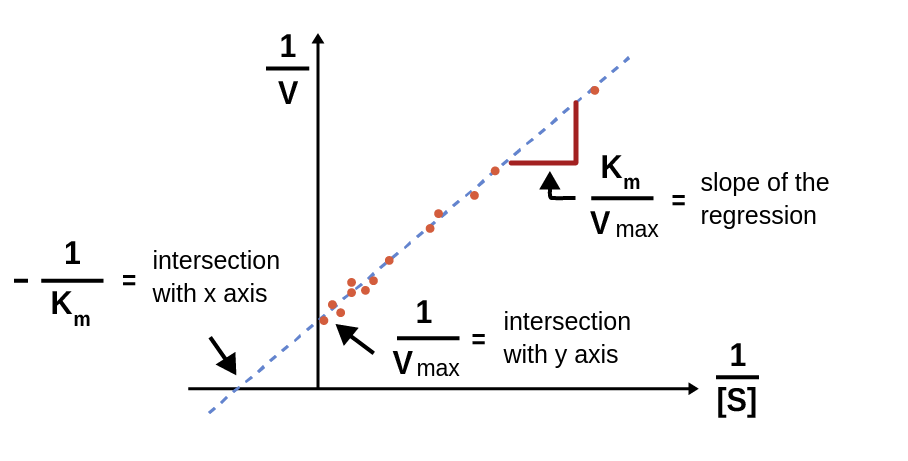In accordance with the Michaelis-Menten equation, the initial reaction rate (V0) increases at increased substrate concentrations [S]. The reaction rate increases more at lower substrate concentrations, and it eventually reaches a plateau, approaching the maximum velocity Vmax (Fig. 1). The maximum initial velocity is reached when the enzyme is saturated, i.e., when enough substrate is present to ensure that practically all the enzyme is part of the enzyme-substrate complex. Because the enzyme can never be completely saturated, Vmax is never fully reached. Vmax is dependent on 2 things: the turnover number of the enzyme (kcat), and the concentration of the enzyme [E] .

Vmax = [E] • kcat

Thus, a higher [E] leads to a higher Vmax. The turnover number will be described in more detail on the following page.Figure 1: An illustration of the Lineweaver-Burk equation fitted to a double-reciprocal transformation of an enzyme kinetic dataset.

## Determining Vmax

Just like km, Vmax can be determined using the Lineweaver-Burk equation (Figure 1):

1/V0 = 1/Vmax + Km/Vmax • 1/[S]

Based on this equation, a straight line fitted to a double reciprocal plot will have the y-intercept 1/Vmax, and Vmax can therefore be obtained by taking the reciprocal to this intercept .

1. Lehninger, Albert L.; Nelson, David L.; Cox, Michael M. (2008). Principles of Biochemistry (5th ed.). New York, NY: W.H. Freeman and Company. ISBN 978-0-7167-7108-1.

kcat

Theory overview